# Electronics and Communication Engineering - Signals and Systems

Exercise : Signals and Systems - Section 1
31.
A signal m(t) is multiplied by a sinusoidal waveform of frequency fc such that
v(t)=m(t) cos 2pfct
If Fourier transform of m(t) is M(f), Fourier transform of v(t) will be
0.5 M(f + fc)
0.5 M(f - fc)
0.5 M(f + fc) + 0.5 M(f - fc)
0.5 M(f - fc) + 0.5 M(f - fc)
Explanation:

It is a modulation process. The resultant has (f + fc) and (f - fc) terms.

32.
A voltage wave having 5% fifth harmonic content is applied to a series RC circuit. The percentage fifth harmonic content in the current wave will be
5%
more than 5%
less than 5%
equal or more than 5%
Explanation:

IC = V(jωC).

33.

Assertion (A): If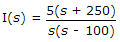, the initial value of i(t) is 5A

Reason (R): As per initial vaue theroem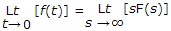Both A and R are correct and R is correct explanation of A
Both A and R are correct but R is not correct explanation of A
A is true, R is false
A is false, R is true
Explanation:

Initial value theorem when applied to I(s) gives 5 A.

34.
δ(t) is a
energy signal
power signal
neither energy nor power
none
Explanation:

Because Unit step is a Power signal. So By trignometric identifies d(t) also power.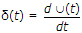.

35.
The analog signal given below is sampled by 600 samples per second for m(t) = 3 sin 500 pt + 2 sin 700 pt then folding frequency is
500 Hz
700 Hz
300 Hz
1400 Hz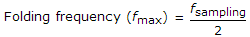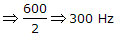.